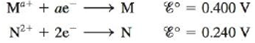# An electrochemical cell is set up using the following unbalanced reaction: M a + ( a q ) + N ( s ) → N 2 + ( a q ) + M ( s ) The standard reduction potentials are: The cell contains 0.10 M N 2+ and produces a voltage of 0.180 V. If the concentration of M a+ is such that the value of the reaction quotient Q is 9.32 × 10 −3 , calculate [M a+ ]. Calculate w max for this electrochemical cell.### Chemistry: An Atoms First Approach

2nd Edition
Steven S. Zumdahl + 1 other
Publisher: Cengage Learning
ISBN: 9781305079243

#### Solutions

Chapter
Section### Chemistry: An Atoms First Approach

2nd Edition
Steven S. Zumdahl + 1 other
Publisher: Cengage Learning
ISBN: 9781305079243
Chapter 17, Problem 156IP
Textbook Problem
1 views

## An electrochemical cell is set up using the following unbalanced reaction: M a + ( a q ) + N ( s ) → N 2 + ( a q ) + M ( s ) The standard reduction potentials are:The cell contains 0.10 M N2+ and produces a voltage of 0.180 V. If the concentration of Ma+ is such that the value of the reaction quotient Q is 9.32 × 10−3, calculate [Ma+]. Calculate wmax for this electrochemical cell.

Interpretation Introduction

Interpretation: The concentration of [Ma+] and wmax of the given electrochemical cell is to be calculated.

Concept introduction: Electrochemical cell is defined as a cell that generates electrical energy from a redox reaction taking place in a cell. Nernst equation used in an electrochemical cell is used to relate the standard reduction potential, reduction potential, activities and temperature in a redox reaction.

To determine: The concentration of [Ma+] and wmax of the given electrochemical cell.

### Explanation of Solution

Explanation

Given

The concentration of N2+ is 0.10 M .

Ecell=0.180 V

Reaction quotient Q 0r[a1]  equilibrium constant is 9.32×103 .

The given reaction is,

Ma++NN2++M

The reaction quotient of the reaction is given as,

[N2+][Ma+]=Q .

The concentration of [Ma+] is calculated as per data,

[N2+][Ma+]=9.32×103 .

The concentration of N2+ is 0.10 M .

Substitute the value of N2+ in the above expression.

[N2+][Ma+]=9.32×103[0.1 M][Ma+]=9.32×103[Ma+]=0

### Still sussing out bartleby?

Check out a sample textbook solution.

See a sample solution

#### The Solution to Your Study Problems

Bartleby provides explanations to thousands of textbook problems written by our experts, many with advanced degrees!

Get Started

Find more solutions based on key concepts
Children who don't drink milk often have lower bone density than than those who do? T F

Nutrition: Concepts and Controversies - Standalone book (MindTap Course List)

7 (Chemical Connections 19C) Name the two functional groups in aspirin.

Introduction to General, Organic and Biochemistry

Write the correct IUPAC name for each of the following: a. c. b. d.

Chemistry for Today: General, Organic, and Biochemistry

A 1 000-kg roller coaster car is initially at the top of a rise, at point . It then moves 135 ft, at an angle o...

Physics for Scientists and Engineers, Technology Update (No access codes included)

Why dont deltas form at every river mouth?

Oceanography: An Invitation To Marine Science, Loose-leaf Versin